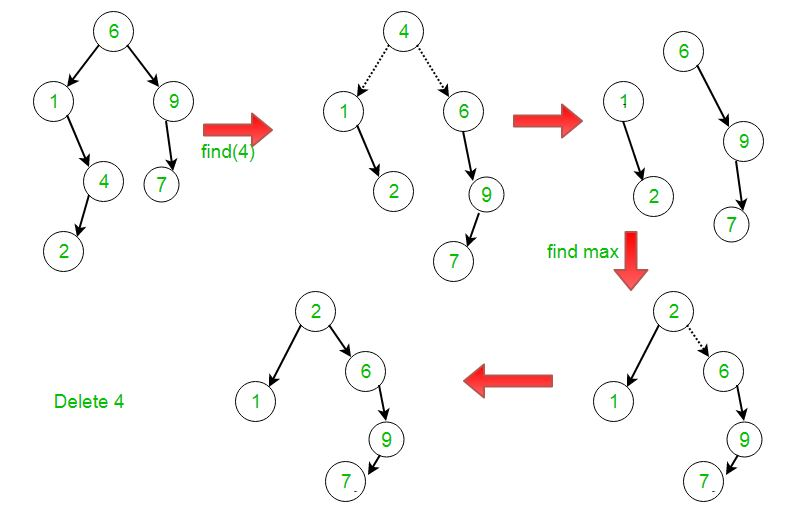GeeksforGeeks App
Open AppBrowser
Continue

# Deletion in Splay Tree

It is recommended to refer following post as prerequisite of this post. Splay Tree | Set 1 (Search) Following are the different cases to delete a key k from splay tree.

1. If Root is NULL: We simply return the root.
2. Else Splay the given key k. If k is present, then it becomes the new root. If not present, then last accessed leaf node becomes the new root.
3. If new root’s key is not same as k, then return the root as k is not present.
4. Else the key k is present.
• Split the tree into two trees Tree1 = root’s left subtree and Tree2 = root’s right subtree and delete the root node.
• Let the root’s of Tree1 and Tree2 be Root1 and Root2 respectively.
• If Root1 is NULL: Return Root2.
• Else, Splay the maximum node (node having the maximum value) of Tree1.
• After the Splay procedure, make Root2 as the right child of Root1 and return Root1.Implementation:

## CPP

 `// C implementation to delete a node from Splay Tree``#include ``#include `` ` `// An AVL tree node``struct` `node {``    ``int` `key;``    ``struct` `node *left, *right;``};`` ` `/* Helper function that allocates a new node with the given``   ``key and NULL left and right pointers. */``struct` `node* newNode(``int` `key)``{``    ``struct` `node* node``        ``= (``struct` `node*)``malloc``(``sizeof``(``struct` `node));``    ``node->key = key;``    ``node->left = node->right = NULL;``    ``return` `(node);``}`` ` `// A utility function to right rotate subtree rooted with y``// See the diagram given above.``struct` `node* rightRotate(``struct` `node* x)``{``    ``struct` `node* y = x->left;``    ``x->left = y->right;``    ``y->right = x;``    ``return` `y;``}`` ` `// A utility function to left rotate subtree rooted with x``// See the diagram given above.``struct` `node* leftRotate(``struct` `node* x)``{``    ``struct` `node* y = x->right;``    ``x->right = y->left;``    ``y->left = x;``    ``return` `y;``}`` ` `// This function brings the key at root if key is present in``// tree. If key is not present, then it brings the last``// accessed item at root. This function modifies the tree``// and returns the new root``struct` `node* splay(``struct` `node* root, ``int` `key)``{``    ``// Base cases: root is NULL or key is present at root``    ``if` `(root == NULL || root->key == key)``        ``return` `root;`` ` `    ``// Key lies in left subtree``    ``if` `(root->key > key) {``        ``// Key is not in tree, we are done``        ``if` `(root->left == NULL)``            ``return` `root;`` ` `        ``// Zig-Zig (Left Left)``        ``if` `(root->left->key > key) {``            ``// First recursively bring the key as root of``            ``// left-left``            ``root->left->left = splay(root->left->left, key);`` ` `            ``// Do first rotation for root, second rotation``            ``// is done after else``            ``root = rightRotate(root);``        ``}``        ``else` `if` `(root->left->key``                 ``< key) ``// Zig-Zag (Left Right)``        ``{``            ``// First recursively bring the key as root of``            ``// left-right``            ``root->left->right``                ``= splay(root->left->right, key);`` ` `            ``// Do first rotation for root->left``            ``if` `(root->left->right != NULL)``                ``root->left = leftRotate(root->left);``        ``}`` ` `        ``// Do second rotation for root``        ``return` `(root->left == NULL) ? root``                                    ``: rightRotate(root);``    ``}``    ``else` `// Key lies in right subtree``    ``{``        ``// Key is not in tree, we are done``        ``if` `(root->right == NULL)``            ``return` `root;`` ` `        ``// Zag-Zig (Right Left)``        ``if` `(root->right->key > key) {``            ``// Bring the key as root of right-left``            ``root->right->left``                ``= splay(root->right->left, key);`` ` `            ``// Do first rotation for root->right``            ``if` `(root->right->left != NULL)``                ``root->right = rightRotate(root->right);``        ``}``        ``else` `if` `(root->right->key``                 ``< key) ``// Zag-Zag (Right Right)``        ``{``            ``// Bring the key as root of right-right and do``            ``// first rotation``            ``root->right->right``                ``= splay(root->right->right, key);``            ``root = leftRotate(root);``        ``}`` ` `        ``// Do second rotation for root``        ``return` `(root->right == NULL) ? root``                                     ``: leftRotate(root);``    ``}``}`` ` `// The delete function for Splay tree. Note that this``// function returns the new root of Splay Tree after``// removing the key``struct` `node* delete_key(``struct` `node* root, ``int` `key)``{``    ``struct` `node* temp;``    ``if` `(!root)``        ``return` `NULL;`` ` `    ``// Splay the given key``    ``root = splay(root, key);`` ` `    ``// If key is not present, then``    ``// return root``    ``if` `(key != root->key)``        ``return` `root;`` ` `    ``// If key is present``    ``// If left child of root does not exist``    ``// make root->right as root``    ``if` `(!root->left) {``        ``temp = root;``        ``root = root->right;``    ``}`` ` `    ``// Else if left child exits``    ``else` `{``        ``temp = root;`` ` `        ``/*Note: Since key == root->key,``        ``so after Splay(key, root->lchild),``        ``the tree we get will have no right child tree``        ``and maximum node in left subtree will get splayed*/``        ``// New root``        ``root = splay(root->left, key);`` ` `        ``// Make right child of previous root as``        ``// new root's right child``        ``root->right = temp->right;``    ``}`` ` `    ``// free the previous root node, that is,``    ``// the node containing the key``    ``free``(temp);`` ` `    ``// return root of the new Splay Tree``    ``return` `root;``}`` ` `// A utility function to print preorder traversal of the``// tree. The function also prints height of every node``void` `preOrder(``struct` `node* root)``{``    ``if` `(root != NULL) {``        ``printf``(``"%d "``, root->key);``        ``preOrder(root->left);``        ``preOrder(root->right);``    ``}``}`` ` `/* Driver program to test above function*/``int` `main()``{``    ``// Splay Tree Formation``    ``struct` `node* root = newNode(6);``    ``root->left = newNode(1);``    ``root->right = newNode(9);``    ``root->left->right = newNode(4);``    ``root->left->right->left = newNode(2);``    ``root->right->left = newNode(7);`` ` `    ``int` `key = 4;`` ` `    ``root = delete_key(root, key);``    ``printf``(``"Preorder traversal of the modified Splay tree "``           ``"is \n"``);``    ``preOrder(root);``    ``return` `0;``}`

Output

```Preorder traversal of the modified Splay tree is
2 1 6 9 7 ```

This article is contributed by Ayush Jauhari. If you like GeeksforGeeks and would like to contribute, you can also write an article using write.geeksforgeeks.org or mail your article to review-team@geeksforgeeks.org. See your article appearing on the GeeksforGeeks main page and help other Geeks.

My Personal Notes arrow_drop_up